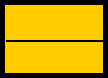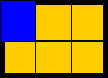Sample Math Lessons1. Placement of the Decimal PointEveryone learns the rule for placing the decimal point, but few can explain the rule.  The rule comes about from pattern recognition and the definition of fractions.  We discovered that when we multiply numbers that are powers of ten, we add the number of zeros in the multiplier and multiplicand to find the number of zeros in the answer:    100 x 1000 = 100,000               2   +    3           5We can write the numbers 1.23  and 1.356 as 123 / 100 and 1356 / 1000, respectively.  It follows from the definition of a fraction that  1 / 100  x  1 / 1000 = 1 / (100 x 1000) = 1 / 100,000Therefore, 1.23  x  1.356  =  123 x 1356 / (100 x 1000)                                           =  166788 / 100,000                                           =  1.66788We can visualize the result of multiplying the denominators by using a manipulative rectangle.  We see that  1/3  x  1/2  =  1 / (3 x 2)  =  1/6.First form halves.           Then form thirds.We can see that the blue part is 1/6 the whole.Pattern recognition makes it easy to remember the rules, putting less emphasis on rote memorization.  A student should be encouraged to question every rule and every explanation.  This challenge is an important part in the learning and thinking process, and this step in learning extends well beyond mathematics.